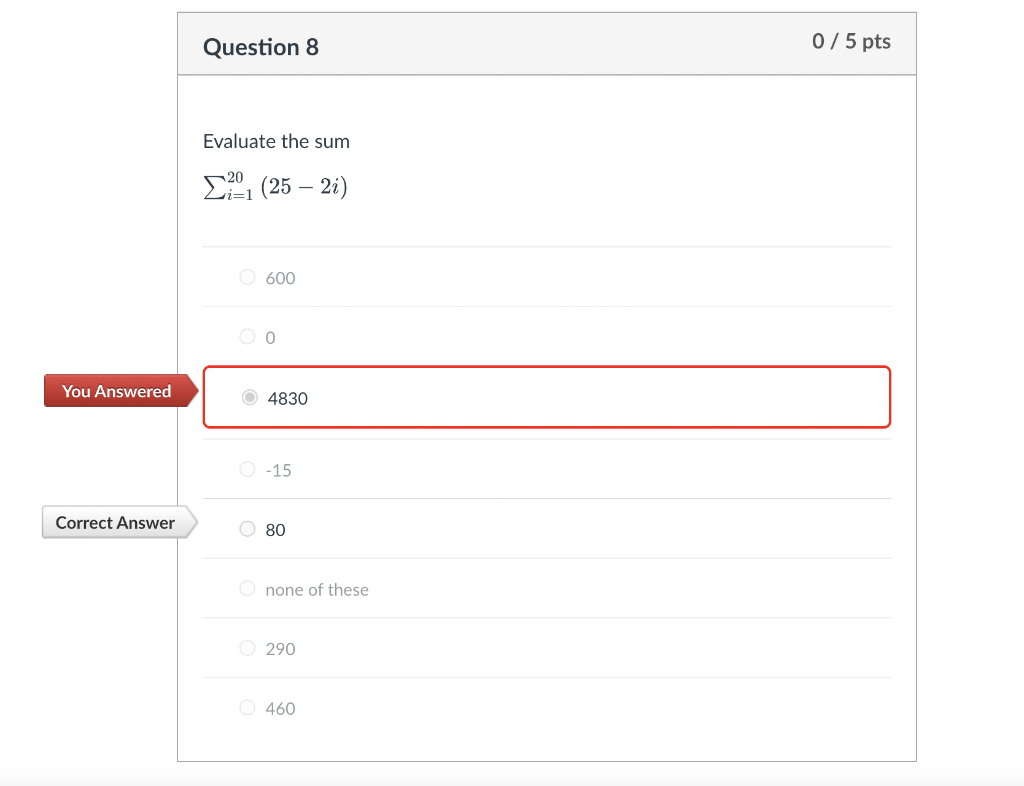Home / Expert Answers / Calculus / evaluate-the-sum-sum-i-1-20-25-2-i-600-0-4830-15-80-none-of-these-290-460-pa257

# (Solved): Evaluate the sum $\sum_{i=1}^{20}(25-2 i)$ 600 0 4830 $$-15$$ 80 none of these 290 460 ...Evaluate the sum $\sum_{i=1}^{20}(25-2 i)$ 600 0 4830 $$-15$$ 80 none of these 290 460

We have an Answer from Expert